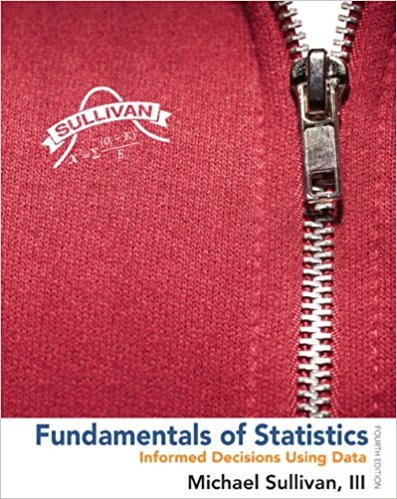×
×

# Solutions for Chapter Chapter 4.1: Fundamentals of Statistics 4th Edition## Full solutions for Fundamentals of Statistics | 4th Edition

ISBN: 9780321838704Solutions for Chapter Chapter 4.1

Solutions for Chapter Chapter 4.1
4 5 0 403 Reviews
16
4
##### ISBN: 9780321838704

This textbook survival guide was created for the textbook: Fundamentals of Statistics, edition: 4. This expansive textbook survival guide covers the following chapters and their solutions. Since 51 problems in chapter Chapter 4.1 have been answered, more than 311212 students have viewed full step-by-step solutions from this chapter. Chapter Chapter 4.1 includes 51 full step-by-step solutions. Fundamentals of Statistics was written by and is associated to the ISBN: 9780321838704.

Key Statistics Terms and definitions covered in this textbook
• a-error (or a-risk)

In hypothesis testing, an error incurred by failing to reject a null hypothesis when it is actually false (also called a type II error).

• Alias

In a fractional factorial experiment when certain factor effects cannot be estimated uniquely, they are said to be aliased.

• Assignable cause

The portion of the variability in a set of observations that can be traced to speciic causes, such as operators, materials, or equipment. Also called a special cause.

• Bayes’ theorem

An equation for a conditional probability such as PA B ( | ) in terms of the reverse conditional probability PB A ( | ).

• Bias

An effect that systematically distorts a statistical result or estimate, preventing it from representing the true quantity of interest.

• Block

In experimental design, a group of experimental units or material that is relatively homogeneous. The purpose of dividing experimental units into blocks is to produce an experimental design wherein variability within blocks is smaller than variability between blocks. This allows the factors of interest to be compared in an environment that has less variability than in an unblocked experiment.

• Box plot (or box and whisker plot)

A graphical display of data in which the box contains the middle 50% of the data (the interquartile range) with the median dividing it, and the whiskers extend to the smallest and largest values (or some deined lower and upper limits).

• Causal variable

When y fx = ( ) and y is considered to be caused by x, x is sometimes called a causal variable

• Central tendency

The tendency of data to cluster around some value. Central tendency is usually expressed by a measure of location such as the mean, median, or mode.

• Contour plot

A two-dimensional graphic used for a bivariate probability density function that displays curves for which the probability density function is constant.

• Control chart

A graphical display used to monitor a process. It usually consists of a horizontal center line corresponding to the in-control value of the parameter that is being monitored and lower and upper control limits. The control limits are determined by statistical criteria and are not arbitrary, nor are they related to speciication limits. If sample points fall within the control limits, the process is said to be in-control, or free from assignable causes. Points beyond the control limits indicate an out-of-control process; that is, assignable causes are likely present. This signals the need to ind and remove the assignable causes.

• Correction factor

A term used for the quantity ( / )( ) 1 1 2 n xi i n ? = that is subtracted from xi i n 2 ? =1 to give the corrected sum of squares deined as (/ ) ( ) 1 1 2 n xx i x i n ? = i ? . The correction factor can also be written as nx 2 .

• Counting techniques

Formulas used to determine the number of elements in sample spaces and events.

• Covariance matrix

A square matrix that contains the variances and covariances among a set of random variables, say, X1 , X X 2 k , , … . The main diagonal elements of the matrix are the variances of the random variables and the off-diagonal elements are the covariances between Xi and Xj . Also called the variance-covariance matrix. When the random variables are standardized to have unit variances, the covariance matrix becomes the correlation matrix.

• Crossed factors

Another name for factors that are arranged in a factorial experiment.

• Cumulative normal distribution function

The cumulative distribution of the standard normal distribution, often denoted as ?( ) x and tabulated in Appendix Table II.

• Defect concentration diagram

A quality tool that graphically shows the location of defects on a part or in a process.

• Deming’s 14 points.

A management philosophy promoted by W. Edwards Deming that emphasizes the importance of change and quality

• Gamma function

A function used in the probability density function of a gamma random variable that can be considered to extend factorials

• Geometric mean.

The geometric mean of a set of n positive data values is the nth root of the product of the data values; that is, g x i n i n = ( ) = / w 1 1 .

×